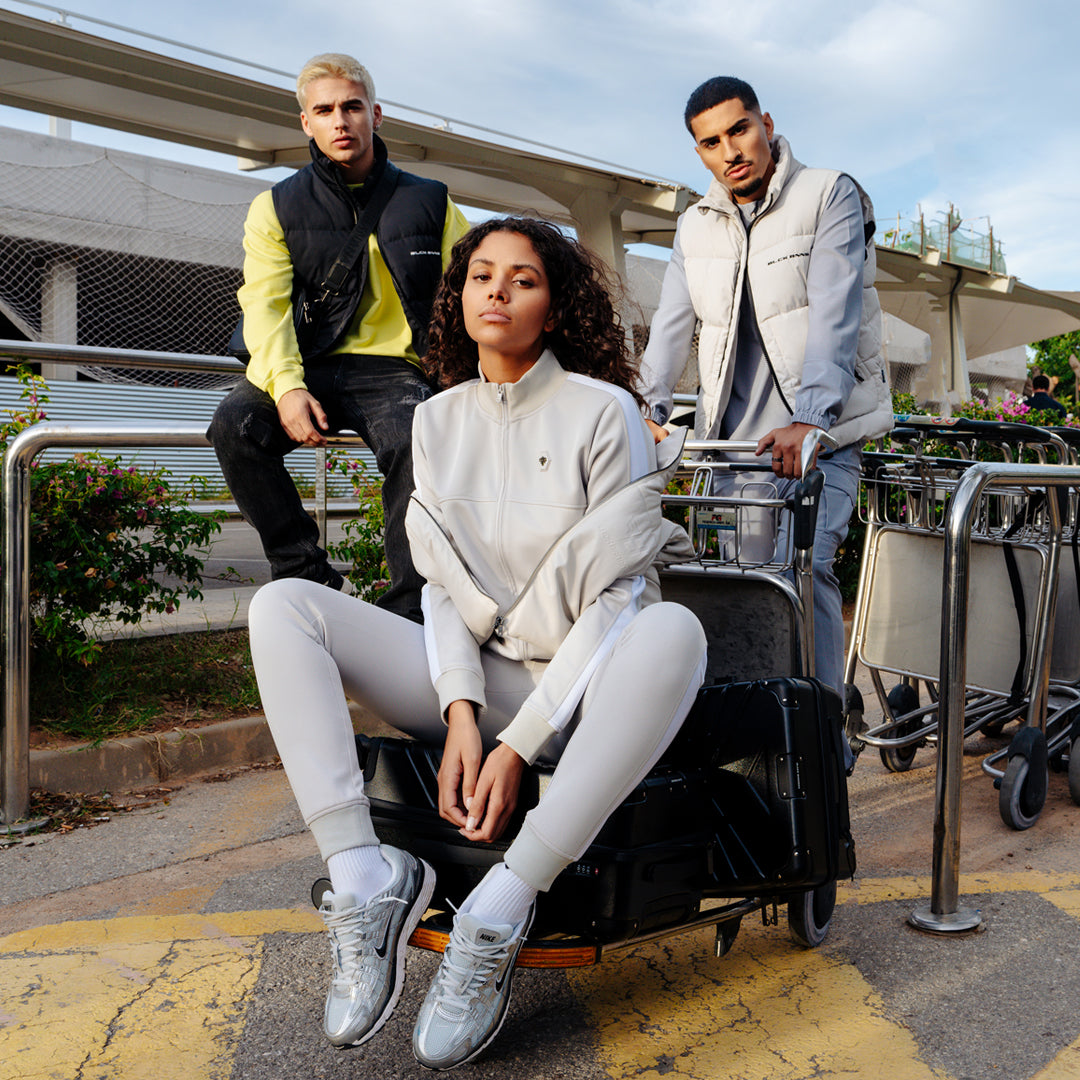• CATCH OUR DEAL OF THE DAY# HEX COLLECTION

The Hex Collection by Black Bananas is a range of high quality and comfortable products. Sports inspired, essential and above all minimalistic styles with a premium branding.

The rubber hexagon logo with the metal Convoy is referring to our sports roots with a fashion touch.

Experience the perfect blend of sporty heritage and sartorial finesse with the Hex Collection by Black Bananas. Immerse yourself in the synergy of fashion-forward minimalism and premium quality that defines each meticulously crafted piece.

The Hex essentials collections contains basic joggers, vests, tees, shorts and hoodies in 7 colorways. Shop 3 essentials and get 25% off.

• XS
• S
• M
• L
• XL
• XXL

• S
• L
• XL
• XXL

• XS
• S
• M
• L
• XL

• XS
• S
• M
• L
• XL

• XS
• S
• M
• L
• XL

• XS
• S
• M
• L
• XL

• S
• M
• L
• XL
• XXL

• L
• XXL

• XS
• S
• M
• L
• XL
• XXL

• XS
• S
• M
• L
• XL
• XXL

• XS
• S
• M
• L
• XL

• XS
• S
• M
• L
• XL

• XS
• S
• M
• L
• XL

• XS
• S
• M
• L
• XL

• XS
• S
• M
• L
• XL
• XXL

• XS
• S
• M
• L
• XL
• XXL

• XS
• S
• M
• L
• XL
• XXL

• One size

• XS
• S
• M
• L
• XL

• XS
• S
• M
• L
• XL

• XS
• S
• M
• L
• XL

• XS
• S
• M
• L
• XL

• XS
• S
• M
• L
• XL
• XXL

• One size

• XS
• S
• M
• L
• XL
• XXL

• XS
• S
• M
• L
• XL
• XXL

• XS
• S
• M
• L
• XL

• XS
• S
• M
• L
• XL

• XS
• S
• M
• L
• XL

• L

• XS
• S
• M
• L
• XL
• XXL

• L
• XL
• XXL

• XS
• S
• M
• L
• XL
• XXL

• One size

• XS
• S
• M
• L
• XL

• XS
• S
• M
• L
• XL

• XS
• S
• M
• L
• XL

• S
• M
• L
• XL

• One size

• XS
• S
• M
• L
• XL
• XXL

• M
• L
• XL
• XXL

• One size

• XS
• S
• M
• L
• XL
• XXL

• M
• L
• XL

• XS
• S
• M
• L
• XL
• XXL

• XS
• S
• M
• L
• XL
• XXL

• XS
• S
• M
• L
• XL

• XS
• S
• M
• L
• XL
• XXL

• One size